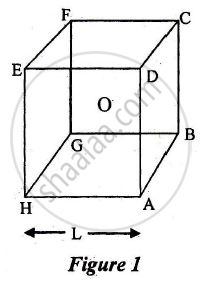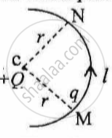# ISC (Arts) Class 12 - CISCE Important Questions for Physics (Theory)

Subjects
Topics
Subjects
Popular subjects
Topics
Physics (Theory)
< prev  1 to 20 of 236  next >

Write two important advantages of reflecting telescope over a refracting telescope.

Appears in 12 question papers
Chapter: [0.061] Ray Optics and Optical Instruments
Concept: Optical Instruments > Telescope

Give the truth table and circuit symbol for NAND gate

Appears in 10 question papers
Chapter: [0.09] Electronic Devices
Concept: Digital Electronics and Logic Gates

Identify the part of the electromagnetic spectrum which is suitable for radar system used in aircraft navigation.

Appears in 6 question papers
Chapter: [0.05] Electromagnetic Waves
Concept: Electromagnetic Spectrum

Draw a labelled ray diagram showing the formation of a final image by a compound microscope at least distance of distinct vision

Appears in 5 question papers
Chapter: [0.061] Ray Optics and Optical Instruments
Concept: Optical Instruments > The Microscope

State de Broglie hypothesis

Appears in 5 question papers
Chapter: [0.07] Dual Nature of Radiation and Matter
Concept: de-Broglie Relation

Derive Snell’s law on the basis of Huygen’s wave theory when the light is travelling from a denser to a rarer medium.

Appears in 2 question papers
Chapter: [0.061] Ray Optics and Optical Instruments
Concept: Snell’s Law

Find the de Broglie wavelength of electrons moving with a speed of 7 × 106 ms -1

Appears in 2 question papers
Chapter: [0.07] Dual Nature of Radiation and Matter
Concept: de-Broglie Relation

On the basis of Bohr's theory, derive an expression for the radius of the nth orbit of an electron of the hydrogen atom.

Appears in 2 question papers
Chapter: [0.081] Atoms
Concept: Bohr’s Model for Hydrogen Atom

Write one balanced equation to show Nuclear fission

Appears in 2 question papers
Chapter: [0.08199999999999999] Nuclei
Concept: Nuclear Energy > Nuclear Fusion – Energy Generation in Stars

What is the use of Zener diode?

Appears in 2 question papers
Chapter: [0.09] Electronic Devices
Concept: Semiconductor Diode

A short electric dipole (which consists of two point charges, +q and -q) is placed at the centre 0 and inside a large cube (ABCDEFGH) of length L, as shown in Figure 1. The electric flux, emanating through the cube is:a) q"/"4piin_9L

b) zero

c) q"/"2piin_0L

d) q"/"3piin_0L

Appears in 1 question paper
Chapter: [0.011000000000000001] Electric Charges and Fields
Concept: Electric Dipole

What is meant by the term Quantization of charge?

Appears in 1 question paper
Chapter: [0.011000000000000001] Electric Charges and Fields
Concept: Quantisation of Charge

Derive an expression for the intensity of electric field at a point in broadside position or on [4)
an equatorial line of an electric dipole.

Appears in 1 question paper
Chapter: [0.011000000000000001] Electric Charges and Fields
Concept: Electric Dipole

Maximum torque acting on an electric dipole of moment  3×10-29  Cm in a uniform electric field E is 6 × 10-25 Nm. Find E.

Appears in 1 question paper
Chapter: [0.011000000000000001] Electric Charges and Fields
Concept: Dipole in a Uniform External Field

The intensity of the electric field at a perpendicular distance of 0·5 m from an infinitely long line charge having linear charge density (λ) is 3-6 × 103 Vm-1. Find the value of λ.

Appears in 1 question paper
Chapter: [0.011000000000000001] Electric Charges and Fields
Concept: Electric Field Lines

In Figure 1 below, a charge Q is fixed. Another charge q is moved along a circular arc MN of radius r around it, from the point M to the point N such that the length of the arc MN = l. The work done in this process is:figure 1

Appears in 1 question paper
Chapter: [0.011000000000000001] Electric Charges and Fields
Concept: Basic Properties of Electric Charge

A charged oil drop weighing 1.6 x 10-15 N is found to remain suspended in a uniform electric field of intensity 2 x 103 Nc-1. Find the charge on the drop.

Appears in 1 question paper
Chapter: [0.011000000000000001] Electric Charges and Fields
Concept: Electric Field Due to a Point Charge

Two-point charges Q1 = 400 μC and Q2 = 100 μC are kept fixed, 60 cm apart in a vacuum. Find the intensity of the electric field at the midpoint of the line joining Q1 and Q2.

Appears in 1 question paper
Chapter: [0.011000000000000001] Electric Charges and Fields
Concept: Electric Dipole

State Gauss’Law.

Appears in 1 question paper
Chapter: [0.011000000000000001] Electric Charges and Fields
Concept: Applications of Gauss’s Law

In an electric dipole, at which point is the electric potential zero ?

Appears in 1 question paper
Chapter: [0.011000000000000001] Electric Charges and Fields
Concept: Electric Dipole
< prev  1 to 20 of 236  next >